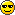# a way to reduce triangles in GeoMipTerrain

if you for some reason use bruteForce on a geomipterrain and use a flat & opaque ocean(or other thing) surface to cover some part of terrain, here is a way to signifacantly reduce triangle count in the terrain.
the basic idea is to remove all triangles which are totally under water. a triangle is totally under water if the color values of all 3 vertices are below a threshold value.
the sample terrain is made of 129x129 heightfield, divided into 16 blocks, 12 blocks are partially under water. there are 24576 triangles in 12 block, this code reduces triangles to 12615, only 51.3% in 24576 are rendered.

``````hf=terrain.heightfield()
tex=Texture()
imgData=tex.getUncompressedRamImage().getData()
terrainNode=terrain.getRoot()
blockSize=int(blockSize)
Xsize,Ysize=hf.getXSize(),hf.getYSize()
blockQty_X,blockQty_Y=(Xsize-1)/blockSize,(Ysize-1)/blockSize
blockToRemove=[block0,block12,block13,block15] # block0 is terrainNode.getChild(0) and so on
for i in range(terrainNode.getNumChildren()):
block=terrainNode.getChild(i)
if block in blockToRemove: continue
hdl=block.node().modifyGeom(0).modifyPrimitive(0).modifyVertices().modifyHandle()
blockID_X=i/blockQty_Y
blockID_Y=i-blockID_X*blockQty_Y
x0=blockID_X*blockSize
y0=blockID_Y*blockSize
triangleQty=blockSize*blockSize*2
old=hdl.getData()
new=''
for j in range(triangleQty):
y1=j-x1*blockSize
left=bool(j<triangleQty/2)
bottom=bool(y1<blockSize/2)
a=x0+x1+(y0+y1)*Xsize
if ord(imgData[a])<threshold and ord(imgData[b])<threshold and ord(imgData[c])<threshold: continue
new+=old[j*6:j*6+6]
if len(new)==0: blockToRemove.append(block); continue
if len(old)!=len(new): hdl.setData(new)
for i in range(len(blockToRemove)): blockToRemove[i].removeNode()``````

I like this, but it raises a question:
What about the gaping holes observable from above the water?

Also I suggest xrange instead of range, for a performance increase.

stackoverflow.com/questions/9493 … python-2-x

thanks for the tip of xrange. i only know basic functions of python.

and what do you mean by gaping holes? the threshold value is chosen to ensure no gap between terrain and water surface.
for example, if posZ of water is 0,
if terrain scaleZ=255, posZ=-6, then threshold=6
if terrain scaleZ=510, posZ=-6, then threshold=3

Terrain and water surface, yeah …
… but water is transparent.

That’s what I am wondering about.

Ofc it’s not an issue if you can’t enter the water or can’t see to the ground.

Just wondering.

this only works for opaque water surface. cheap graphics, low requirement application.the camera must not enter water.

That makes sense.